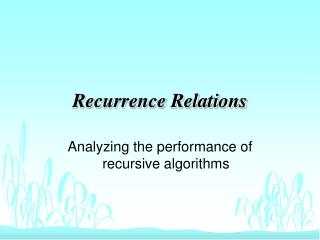Download PresentationRecurrence RelationsRecurrence Relations - PowerPoint PPT Presentation

Download PresentationRecurrence Relations
An Image/Link below is provided (as is) to download presentation

Download Policy: Content on the Website is provided to you AS IS for your information and personal use and may not be sold / licensed / shared on other websites without getting consent from its author. While downloading, if for some reason you are not able to download a presentation, the publisher may have deleted the file from their server.

- - - - - - - - - - - - - - - - - - - - - - - - - - - E N D - - - - - - - - - - - - - - - - - - - - - - - - - - -
Presentation Transcript

1. Recurrence Relations Analyzing the performance of recursive algorithms

2. Worst Case Analysis • Select a Hypothetical Set of Inputs • Should be expandable to any size • Make Algorithm run as long as possible • Must not depend on Algorithm Behavior • May use static behavior analysis • Inputs may not change dynamically

3. Average Case Analysis • Determine the range of inputs over which the average will be taken • Select a probability distribution • Characterize Amount of work required for each input • Compute the Mean Value

4. Binary Search BinS(X:Item;First,Last:Integer):Integer var Middle:Integer; begin if First <= Last then Middle = (First + Last)/2; if L[Middle] = X then return Middle; if L[Middle] < X then return BinS(X,Middle+1,Last); if L[Middle] > X then return BinS(X,First,Middle-1); else return -1; endif; end;

5. Analysis of BinS: Basis • If List Size = 1 then First = Last • If List Size = 0 then First > Last if First <= Last then ... else return -1; • Then W(0) = 0

6. Analysis of BinS: Recurrsion I • If comparison fails, find size of new list • If list size is odd: • First=1,Last=5,Middle=(1+5)/2=3 • If list size is even: • First=1,Last=6,Middle=(1+6)/2=3

7. Analysis of BinS: Recurrsion II • Exercise: Verify that these examples are characteristic of all lists of odd or even size • Worst case: Item X not in list, larger than any element in list. • If list is of size n, new list will be of size:

8. Analysis of BinS: Recurrence Is W(n) ÎQ(n)?

9. Solving the Recurrence We need to eliminate W from the RHS.

10. Continuing the Exercise ...

11. Ah Ha! A General Formula!

12. Ah Ha! A General Formula! But, if k is big enough ...

13. Ah Ha! A General Formula! But, if k is big enough ... Then the argument of W will be zero, and ...

14. Ah Ha! A General Formula! But, if k is big enough ... Then the argument of W will be zero, and ... Since W(0) = 0 ...

15. Ah Ha! A General Formula! But, if k is big enough ... Then the argument of W will be zero, and ... Since W(0) = 0 ... The W on the RHS ...

16. Ah Ha! A General Formula! But, if k is big enough ... Then the argument of W will be zero, and ... Since W(0) = 0 ... The W on the RHS ... Will Disappear!

17. When Will W Disappear? When: Take the Log:

18. When Will W Disappear? When: Take the Log: Take the Floor:

19. Finally ...

20. Some Other Recurrences

21. Recurrences with T(1)=1

22. The Challenger

23. The Master Theorem I

24. The Master Theorem II

25. The Master Theorem III (for sufficiently large n)

26. Homework • Page 72, 4-1 • Page 73, 4-4